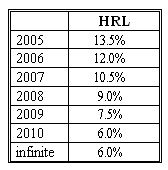### CFA Practice Question

There are 290 practice questions for this topic.

### CFA Practice Question

Beth is evaluating HRL Co. which has been growing at the annual rate of 15% for the last 5 years. Here is her forecast of the company's future dividend growth trend.After considerable study, she has decided to use the following information in her valuation (as of the end of 2004):

• Beta: 1.2.
• 2004 dividend: \$5.
• The risk-free rate: 4%.

What is the value of the HRL's stock?
Correct Answer: The forecast shows that it converges linearly from last year's growth rate of 15% to the mature growth rate of 6% during 2005 - 2010 (the transition period is 6 years, therefore H=3). The required rate of return on HRL stock is rHRL = rf + beta x market premium = 0.04 + 1.2 x 0.06 = 0.112, or 11.2%.

Using the formula for the H-model, we value the HRL company:

VHRL2004 = [D2004 (1 + gL) + D2004 H (gS -gL)] / (r - gL) = [5 x (1 + 0.06) + 5 x 3 x (0.15 - 0.06)] / (0.112 - 0.06) = \$127.88.

User Comment
tengo the growth rate after the first year of decline is 13.5% . It was growing 15% before that time. That % is in the narrative not the table. A bit tricky.
Lavay The last 5 yrs statement in the narrative could be confused for H, whislt the actual transition period is (2005-2010=6 yrs).
jhmorris Am I missing something here? Rather than using the H-model, I simply set up the cash flows (as listed below) and discounted them back at 11.2% to generate a value of \$123.86. Could this simply be due to the approximate nature of the H-model?

CF 1 (2005) = 5.675
CF 2 (2006) = 6.356
CF 3 (2007) = 7.02338
CF 4 (2008) = 7.6555
CF 5 (2009) = 8.2296
CF 6 (2010) = 8.7234 + 177.8231*

* Terminal Value = 9.246804 / (0.112 - 0.06)
Rotigga I did the exact same work as jhmorris and arrived at \$123.86; remembering the H-model formula will probably save some time on the exam though
creativemny In order to use the H-Model the decline of supernormal growth is assumed to be linear. Not sure how this question indicates use of the H-Model.
gaai By starting with 13,5% you get 123.56 which is much closer to the "actual number" 123.86
The 15% is just a history and then Do = 4.35
davidt876 i screwed this up and then looked into it. the question is right, and we should use 15% because it's the current growth rate, and \$5 as it's the current dividend.

and i think i figured out why the valuations are so different. we are treating the dividends paid from 2004-2010 as a continuum, and not as distinct payments at t=1, t=2... basically we are valuing the dividends as if they were spread equally throughout the year - and not paid at the end of the year. time value of money says if we get paid sooner it increases PV, so H-Model produces a higher PV. and in my opinion it's an artificially higher PV.

but there is logic to it because we're treating the abnormal growth period in the same way we do the constant growth period under GGM.

if you really are interested in seeing the logic behind the H-model, go on excel and enter the below data:

A(yr) B(r) C(g)
2004 1.12 1.150
2005 1.12 1.135
2006 1.12 1.105
2007 1.12 1.090
2008 1.12 1.075
2009 1.12 1.060
2010 1.12 1.060
2011 1.12 1.060

plot the above on a line graph and you should see the straight line of r, crossing the ‾\_ looking line of g.

GGM formula:

V2010 = D2010 * (1+g) / (r-g)

if we abstract the above formula while looking at the graph we could say that V2010 is equal to D2010 multiplied by the area under the line 1+g, divided by the area between 1+r and 1+g.

area under line 1+g = height*base = (1+g)*infinity, divided by
area between 1+r and 1+g = height*base = [(1+r)-(1+g)]*infinity = (r-g)*infinity

base = infinity because it's a perpetuity. so:

V2010 = D2010 * (1+g)*infinity / (r-g)*infinity or:
V2010 = D2010 * (1+g)/(r-g) * infinity/infinity

so it's still GGM because infinity/infinity = 1.

now we can agree that the GGM can be abstracted as the area under 1+g divided by the area between r-g. so we look back before 2010, and figure we can calculate the area under 1+g all the way back to 2004 and divide it by area under r-g.

GGM value of constant growth starting from 2004:

V2004 = 5*(1.06)/(0.112-0.06) = 101.92

now we need to add the GGM estimation of the abnormal growth which forms a triangle above the rectangle of constant growth. the area is 0.5*base*height:

base = 2010 - 2004 = 6
height = (1+gS) - (1+gL) = gS - gL = 0.15-0.06 = 0.09

Value of abnormal growth from 2004 to 2010:

V2004 = D2004 * (0.5*base*height) / (r-g) = 5 * (0.5*6*0.09)/(0.112-0.06)
V2004 = 25.96

Add them up = 101.92 + 25.96 = 127.88
ashish100 ^ this guy is on some super potent ADHD medicine if I had to bet. Who has time to type a research report in the comments. Don't even read it coz its so long.

CFA should hire him write their material. Probably would make it even longer lol jaisus